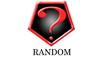random 订阅
random即随机数发生器，使用之前需要使用Randomize语句进行随机数种子的初始化。RANDOM产生的是伪随机数或者说是用一种复杂的方法计算得到的序列值，因此每次运算时需要一个不同的种子值。种子值不同，得到的序列值也不同。因此也就是真正的随机数了。这也正是RANDOMIZE随机初始化的作用。 VB里用 NEW RANDOM()来表示初始化。 展开全文random即随机数发生器，使用之前需要使用Randomize语句进行随机数种子的初始化。RANDOM产生的是伪随机数或者说是用一种复杂的方法计算得到的序列值，因此每次运算时需要一个不同的种子值。种子值不同，得到的序列值也不同。因此也就是真正的随机数了。这也正是RANDOMIZE随机初始化的作用。 VB里用 NEW RANDOM()来表示初始化。

random

nt random(int num)
random基本介绍

• random
千次阅读
2021-03-04 05:46:55

random

java中我们有时候也需要使用使用random来产生随机数，下面我来简单的介绍下java中random的使用方法

public static double random()

返回带正号的 double 值，该值大于等于 0.0 且小于 1.0。返回值是一个伪随机选择的数，在该范围内(近似)均匀分布。

第一次调用该方法时，它将创建一个新的伪随机数生成器，与以下表达式完全相同

new java.util.Random

之后，新的伪随机数生成器可用于此方法的所有调用，但不能用于其他地方。

此方法是完全同步的，可允许多个线程使用而不出现错误。但是，如果许多线程需要以极高的速率生成伪随机数，那么这可能会减少每个线程对拥有自己伪随机数生成器的争用。

返回：

大于等于 0.0 且小于 1.0 的伪随机 double 值。public classRandomDemo {public static voidmain(String[] args) {//创建一个Math.random()的对象

double random =Math.random();//输出的值是[0.0-1.0)之间的随机数

System.out.println(random);/** 输出的结果

* 第一次：0.8302600019925631

* 第二次：0.27576570444589144

* ...

**/}

}

第二种：random()

Random()：创建一个新的随机数生成器。

Random(long seed)：使用单个 long 种子创建一个新的随机数生成器。

Random

更多相关内容
• ## random函数

千次阅读 2021-04-21 22:53:09
手机评站网今天精心准备的是《random函数》，下面是详解！random函数的用法是turbopascal中的函数，希望有具体的介绍(有程序最好)...是turbo pascal 中的函数，希望有具体的介绍(有程序最好)用法：1、随机生成(0,1)...

手机评站网今天精心准备的是《random函数》，下面是详解！

random函数的用法

是turbopascal中的函数，希望有具体的介绍(有程序最好)...

是turbo pascal 中的函数，希望有具体的介绍(有程序最好)

用法：

1、随机生成(0,1)之间的浮点数

random.random()

2、随机生成100-200的整数

random.randint(100,200)

3、随机产生范围为10间隔为2的数

random.randrange(0,11,2)

注：这里输出(0,2,4,6,8,10)中一个

4、从序列中随机抽选一个数

random.choice(list)

5、随机排序

random.shuffle(list)

注：list元素为数值型

从序列中获取指定长度为3的字符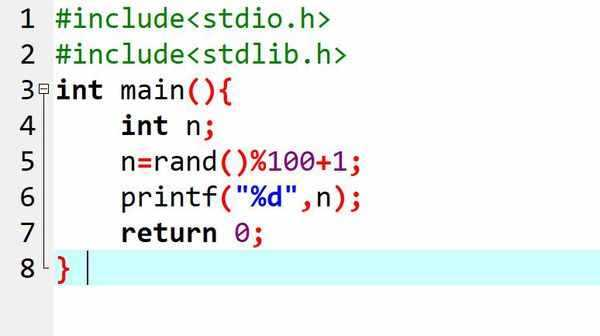扩展资料：

Python的random方法

random.random()用于生成一个指定范围内的随机符点数，两个参数其中一个是上限，一个是下限。如果a > b，则生成随机数 n: a <= n <= b。如果 a

print random.uniform(10, 20) print random.uniform(20, 10)

#18.7356606526

#12.5798298022

random.randint() 用于生成一个指定范围内的整数。其中参数a是下限，参数b是上限，Python生成随机数

print random.randint(12, 20) #生成的随机数n: 12 <= n <= 20 print random.randint(20, 20) #结果永远是20

print random.randint(20, 10) #该语句是错误的。

下限必须小于上限。

c语言里 random函数怎么实现的

他是怎么从随机数里随意选的一个数，想知道他实现的过程，...

他是怎么从随机数里随意选的一个数，想知道他实现的过程，

rand函数是根据一个数(我们可以称它为种子)为基准，以某个递推公式推算出来的一系数，当这系列数很大的时候，就符合正态公布，从而相当于产生了随机数，

但这产生的并不是真意正义上的随机数，是一个伪随机数，当计算机正常开机后，这个种子的值是定了的，除非你破坏了系统，为了改变这个种子的值。

种子相同，产生的随机序列相同。这样做的好处是，方便我们产生一组固定的随机序列，用来调试程序。

C提供了srand()函数，用来设置种子，它的原形是void srand( int a)。

在调用rand函数产生随机数前，应该先利用srand()设好随机数种子，如果未设随机数种子，默认种子为1。

c++中的RANDOM()函数怎么使用?

我想取1-100之间的整数!为什么作了一个10次的循环,用random输出的都是一个数呀?是不是应该输出10个不同的数呀??我刚学习C++不懂,谢谢大家能帮我做详细解答!#include#includ...

我想取1-100之间的整数!

为什么作了一个10次的循环,用random输出的都是一个数呀?是不是应该输出10个不同的数呀??我刚学习C++不懂,谢谢大家能帮我做详细解答!

#include

#include

#include

using namespace std;

int main()

{

for (int i=0;i<10;i++)

{

randomize();

unsigned int r=random(100);

cout<

}

return 0;

}

是这个样子吗??不行呀??

展开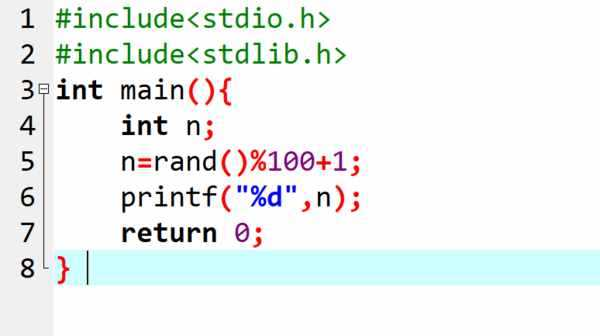方法：

rand()函数用来产生随机数，但是，rand()的内部实现是用线性同余法实现的，是伪随机数，由于周期较长，因此在一定范围内可以看成是随机的。

rand()会返回一个范围在0到RAND_MAX(32767)之间的伪随机数(整数)。

在调用rand()函数之前，可以使用srand()函数设置随机数种子，如果没有设置随机数种子，rand()函数在调用时，自动设计随机数种子为1。随机种子相同，每次产生的随机数也会相同。

rand()函数需要的头文件是：

rand()函数原型：int rand(void);

使用rand()函数产生1-100以内的随机整数：int number1 = rand() % 100;

C++中的rand( )应该小写，需要加载头文件

拓展资料

rand( )函数不是真正的随机数生成器，而srand()会设置供rand()使用的随机数种子。如果你在第一次调用rand()之前没有调用srand()，那么系统会为你自动调用srand()。而使用同种子相同的数调用 rand()会导致相同的随机数序列被生成。

Python里的random.random函数包括0.0和1.0在内吗

你可以查询一下api (帮助文档) 在api 中写道 Math.random() 函数返回的值 大于等于0.0小于1.0所以包括0 不包括1

js里面random()函数问题

Math.random()*3这样能输出0和3这两头的数吗？试了好久都是0到3中间的数...

Math.random()*3

这样能输出0和3这两头的数吗？

试了好久都是0到3中间的数

Math.random()的返回值是个纯小数，即0到0.999999...之间的数，把它再乘以3以后就是0到2.999999...，所以要想得到0到3(包括0和3)的整数，必须这样：

parseInt(Math.random()*4)

Verilog中的&random系统函数

我在编写一个testbench的时候需要产生一个2进制的随机序列，代码中有句为：in={$random}%2;但是编译的时候报错：Error(10174):VerilogHDLUnsupportedFeatureerrorattop_tb.v(22):syst... 我在编写一个testbench的时候需要产生一个2进制的随机序列，代码中有句为： in= {$random} %2;

但是编译的时候报错：Error (10174): Verilog HDL Unsupported Feature error at top_tb.v(22): system function "$random" is not supported for synthesis 请问这是什么原因？ timescale 1 ps/ 1 ps module top_tb(); reg in,en,clk; lpm_shift tb (.clk (clk), .en( en ),.in ( in ), .q ( q )); initial begin in=0; en=1; end always #50 clk = ~clk; always@ (posedge clk) in= {$random} %2;

endmodule

展开

{\$random} 这个语句是不能综合的，只能作为仿真来使用，一般都用在testbench里面，仿真的话这个程序用modelsim是可以通过的。要想产生可综合的程序，楼主可以研究下伪随机序列的算法，使用fpga实现。

matlab中random函数的用法

具体使用方法...

具体使用方法

matlab中random函数——通用函数，求各分布的随机数据，其用法：

y = random('norm',A1,A2,A3,m,n)

式中：A1，A2，A3为分布的参数，m，n用来指定随机数的行和列，name的取值有相关的表格来参照。

例：产生一个3行4列均值为2、标准差为0.3的正态分布随机数：

>>y =random('norm',2,0.3,3,4)

y =

2.1613 2.2587 1.8699 2.8308

2.5502 2.0956 2.1028 1.5950

1.3223 1.6077 3.0735 2.9105

关于VB中的random函数

'初始化随机数初值,得到一个0~9内的随机整数Randomizem_intCurrentConnodity=Int(10*Rnd)在这个函数中得到一个随机整数,但是当程序再次调用此函数的时候,产生的数与刚刚产生的一样?请...

'初始化随机数初值,得到一个0~9内的随机整数

Randomize

m_intCurrentConnodity = Int(10 * Rnd)

在这个函数中得到一个随机整数,但是当程序再次调用此函数的时候,产生的数与刚刚产生的一样?

请问怎样才能让它真正的随机啊,就是当程序每次调用它的时候都能生成一个新的随机数~

展开

反之，有些时候伪随机数的精确重复也是有用的。这可以在一个随机系列开始前，通过调用一个使用固定数值的randomSeed()函数来完成。

有关C语言中random函数的用法

#include#includemain(){inti,j;srand(100);for(i=0;i<10;i++){j=rand();printf("%d",j);}}这段程序实现的是什么？并且怎么用这个函数产生1到100之间的随机数？最...

#include

#include

main()

{

int i,j;

srand(100);

for(i=0;i<10;i++)

{

j=rand();

printf("%d ",j);

}

}

这段程序实现的是什么？并且怎么用这个函数产生1到100之间的随机数？最好再给个例子。谢绝复制！！

展开

这段程序实现是产生十个随机数，产生1到100之间的随机数可以通过mod100+1来实现，代码如下：

#include

#include

main()

{

int j;

srand(100);

j=rand()%100+1;

}

JAVA中如何使用Random函数，该使用那些类？

JAVA中如何使用Random函数，该使用那些类？具体如何吊用？...

JAVA中如何使用Random函数，该使用那些类？具体如何吊用？

Random random = new Random();

Random random = new Random(100);//指定种子数100

method：nextInt()、nextLong()、nextFloat()、nextDouble() --》可以获得不同类型的随机数

如------->System.out.println(random1.nextInt());

//java.lang.Math中的方法Random() 只产生double类型的随机数

你想用它产生0-10的随机数可以这样--->(int)(Math.random()*10);

展开全文• random np.random random() 生成一个[0, 1.)之间的随机浮点数 random([3, 2]) 生成形状为3×2的[0, 1.)之间的随机浮点数 rand(3, 2) 和random([3, 2])相同 randint(a ,b, size) 生成[a,b)...

## random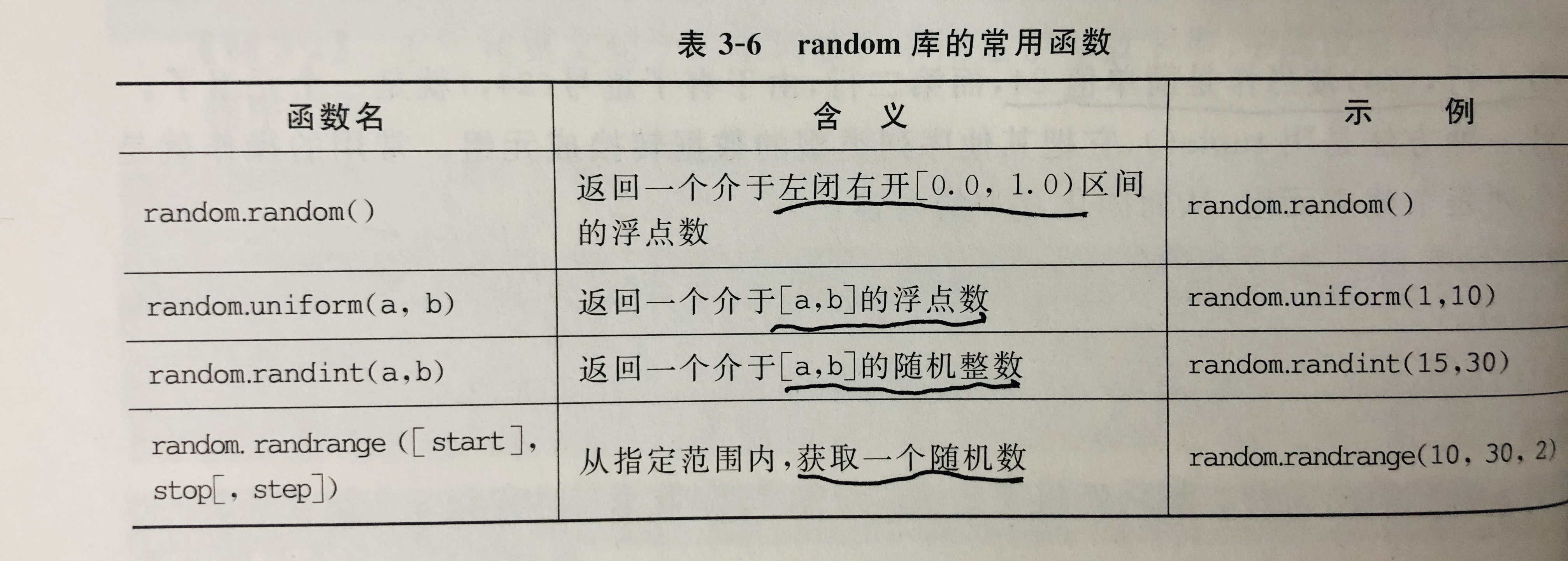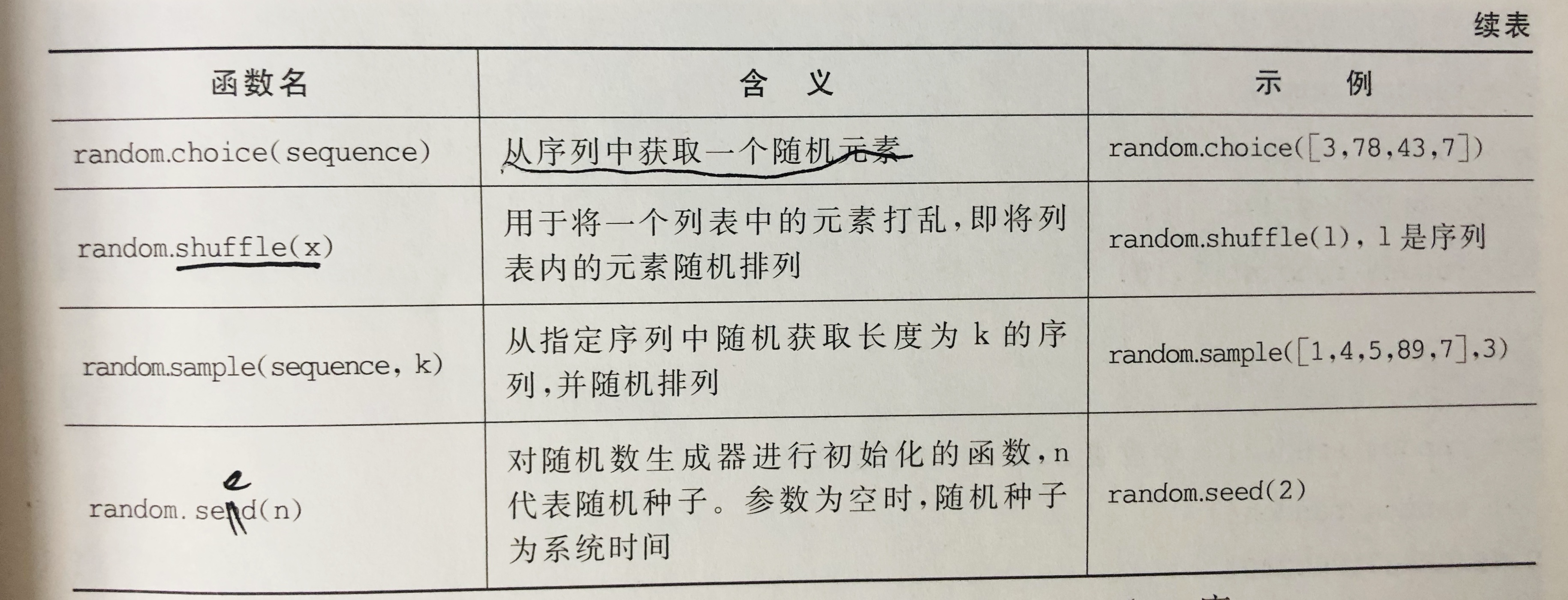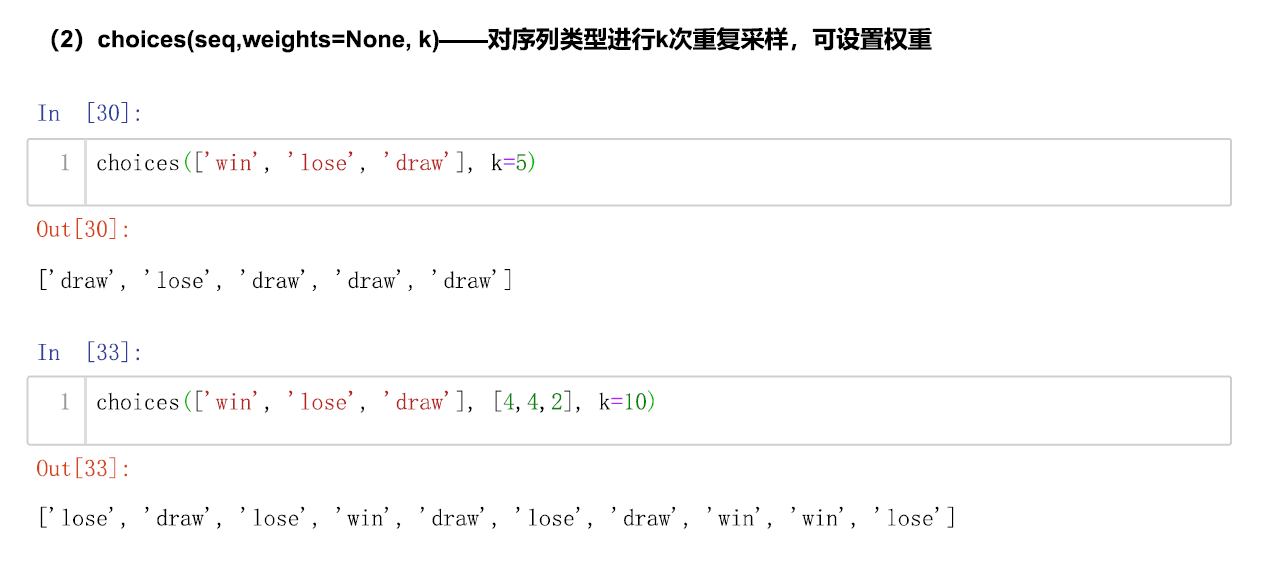## np.random

 random() 生成一个[0, 1.)之间的随机浮点数 random([3, 2]) 生成形状为3×2的[0, 1.)之间的随机浮点数 rand(3, 2) 和random([3, 2])相同 randint(a ,b, size) 生成[a,b)之间的随机整数 uniform(a, b, size) 生成形状为size的[a,b)之间的浮点数 normal(m, d, size) 生成的随机数符合均值为m,标准差为d的正态分布 randn(2, 3) 生成形状为2×3的随机浮点数,符合标准正态分布 choice(l, size) 随机返回形状为size的元素,l必须是1维的 shuffle(arr) 将ndarray中元素随机打乱,直接改变原来的变量,无返回值

1.np.random.random(size)：生成[0, 1.0)之间的随机浮点数。

import numpy as np
'''
np.random.random(size)
Args:
size:默认为None，表示生成随机数的形状，不传参数时，只返回一个随机数
'''
r = np.random.random()
print(r)
'''
0.051330718987810275
'''

r = np.random.random([3,2])
print(r)
'''
[[0.56485029 0.26826023]
[0.283601   0.06587369]
[0.53037703 0.09549962]]
'''

2.np.random.rand(d0, d1, ..., dn)： 生成一个[0,1)之间的随机浮点数或N维浮点数组。

3.np.random.randint(low, high, size):生成[low, high)之间的随机整数。

import numpy as np
'''
np.random.randint(low, high, size)
生成[low,high)之间的整数
Args:
low:最小值(能取到)
high:最大值(取不到)
size:默认为None，表示生成随机数的形状，不传参数时，只返回一个随机数
'''
r = np.random.randint(1, 6)
print(r)
'''
5
'''

r = np.random.randint(1, 7, [3, 2])
print(r)
'''
[[1 3]
[1 6]
[2 2]]
'''

4.np.random.uniform(low, high, size):生成[low,high)之间的随机浮点数，随机的概率是均匀的。

import numpy as np
'''
np.random.uniform(low, high, size)
生成[low,high)之间的随机浮点数，随机的概率是均匀的
Args:
low:最小值(能取到)
high:最大值(取不到)
size:默认为None，表示生成随机数的形状，不传参数时，只返回一个随机数
'''
r = np.random.uniform(1, 6)
print(r)
'''
4.012983373039803
'''

r = np.random.uniform(1, 6, [3, 2])
print(r)
'''
[[3.15760289 1.680493  ]
[5.99482432 3.50459819]
[3.60929118 1.36948006]]
'''

5.np.random.normal(mean, stddev, size):生成形状为size的随机浮点数，随机的概率符合均值为mean，标准差为stddev的正态分布。

import numpy as np
'''
np.random.normal(mean, stddev, size)
生成形状为size的随机浮点数，随机的概率符合均值为mean，标准差为stddev的正态分布
Args:
mean:均值，默认为0
stddev:标准差，默认为1
size:默认为None，表示生成随机数的形状，不传参数时，只返回一个随机数
'''
r = np.random.normal(5, 1)
print(r)
'''
4.881012596562961
'''

r = np.random.normal(5, 1, [3, 2])
print(r)
'''
[[5.28539653 6.53396064]
[5.59970247 5.066448  ]
[5.72564169 4.78830441]]
'''

6.np.random.randn(d0, d1, ..., dn)： 生成一个浮点数或N维浮点数组，取数范围：正态分布的随机样本数。

7.np.random.choice(a, size=None, replace=True, p=None)：从a序列中随机取出形状为size的元素。

import numpy as np
'''
np.random.choice(a, size=None, replace=True, p=None)
从a序列中随机取出形状为size的元素
Args:
a:一个列表，必须是一维的！
size:默认为None，表示生成随机数的形状，不传参数时，只返回一个随机数
replace:能否重复
p=None
'''
r = np.random.choice([1, 2, 3, 4, 5, 6])
print(r)
'''
3
'''

r = np.random.choice([1, 2, 3, 4, 5, 6], [2, 3])
print(r)
'''
[[4 4 6]
[2 2 2]]
'''

r = np.random.choice([1, 2, 3, 4, 5, 6], [2, 3], replace=False)  # 不重复
print(r)
'''
[[3 1 2]
[5 4 6]]
'''

r = np.random.choice([1, 2, 3, 4, 5, 6], [2, 3], p=[0.8, 0.05, 0.05, 0.04, 0.04, 0.02])  # 指定概率
print(r)
'''
[[6 1 1]
[3 1 1]]
'''

8.np.random.shuffle(x)：洗牌，对x进行顺序打乱，对多维数组进行打乱排列时，只对第一个维度也就是列维度进行随机打乱。

import numpy as np
'''
np.random.shuffle(x)
洗牌，对x进行顺序打乱
对多维数组进行打乱排列时，只对第一个维度也就是列维度进行随机打乱
Args:
x：如果是1维的，则全部打乱，如果是多维的,只对第一维打乱
'''
r = np.random.randint(1, 7, 6)  # 一维的
print(r)
'''
[4 5 2 5 1 2]
'''

np.random.shuffle(r)  # 打乱
print(r)
'''
[2 1 4 5 2 5]
'''

r = np.random.randint(1, 7, [5, 2])  # 多维的
print(r)
'''
[[2 1]
[4 6]
[3 2]
[4 1]
[1 4]]
'''

np.random.shuffle(r)  # 打乱
print(r)
'''
[[4 1]
[2 1]
[4 6]
[3 2]
[1 4]]
'''

9.np.random.choice(a, size=None, replace=True, p=None)： 从序列中获取元素，若a为整数，元素取值为np.range(a)中随机数；若a为数组，取值为a数组元素中随机元素。

10.np.random.permutation(x)： 与numpy.random.shuffle(x)函数功能相同，两者区别：peumutation(x)不会修改X的顺序。

## torch随机函数

1.torch.rand(*sizes) ，size可以是列表、元组或直接写数字

Returns a tensor filled with random numbers from a uniform distribution on the interval [0, 1)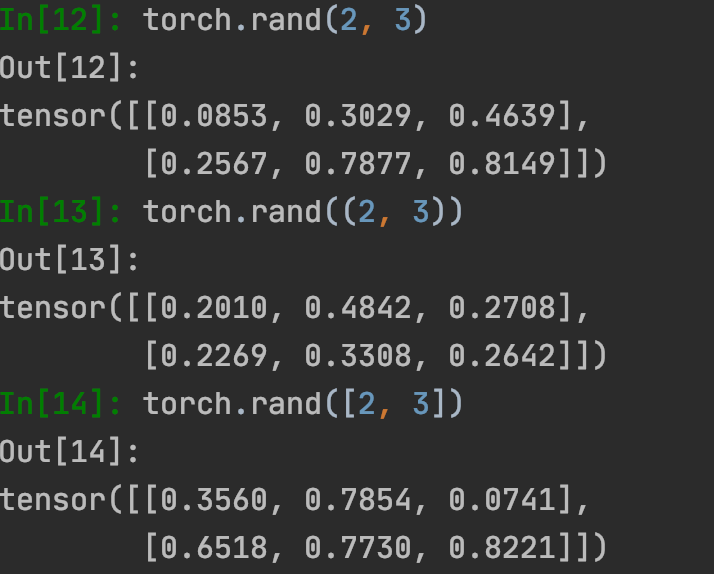2.torch.randn(*sizes) ，size可以是列表、元组或直接写数字

Returns a tensor filled with random numbers from a normal distribution with mean 0 and variance 1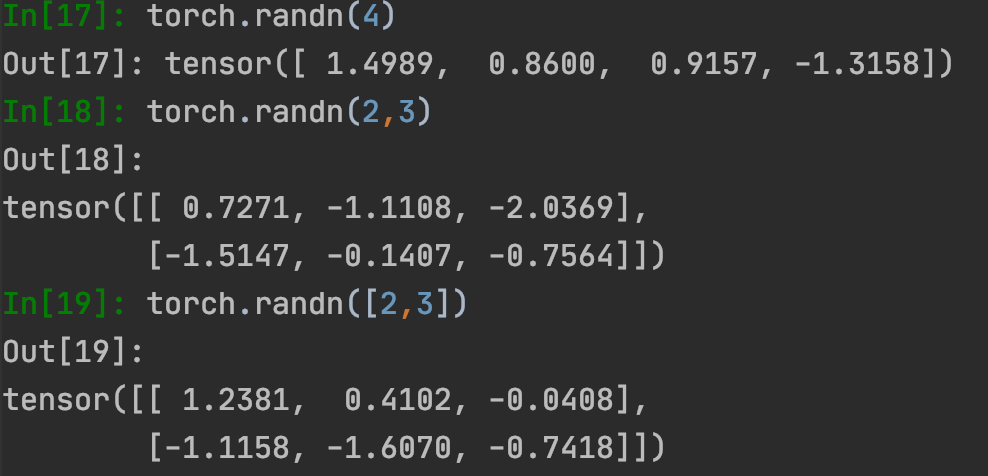3.torch.randint(low, high, size)，size是元组形式

Returns a tensor filled with random integers generated uniformly on the interval [low, high)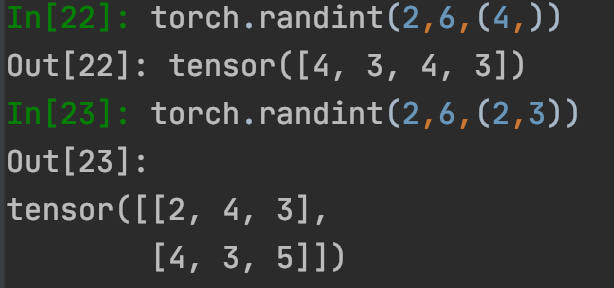4.torch.randperm()：返回一个0到n-1的数组。

5.random_()：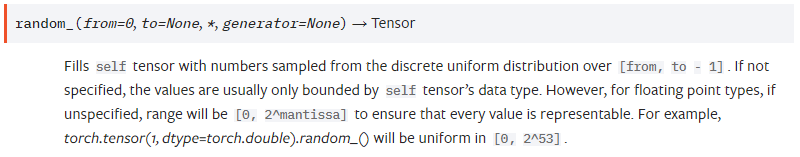返回的是恰好为整数的浮点型数字，例如：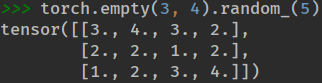展开全文python
• 1. Math.random() 静态方法 产生的随机数是 0 - 1 之间的一个 double，即 0 <= random <= 1。 for (int i = 0; i < 10; i++) { System.out.println(Math.random()); } 结果： 0.3598613895606426 0....

# 1. Math.random() 静态方法

产生的随机数是 0 - 1 之间的一个 double，即 0 <= random <= 1。

for (int i = 0; i < 10; i++) {
System.out.println(Math.random());
}


结果：

0.3598613895606426

0.2666778145365811

0.25090731064243355

0.011064998061666276

0.600686228175639

0.9084006027629496

0.12700524654847833

0.6084605849069343

0.7290804782514261

0.9923831908303121

实现原理：

When this method is first called, it creates a single new pseudorandom-number generator, exactly as if by the expression new java.util.Random() This new pseudorandom-number generator is used thereafter for all calls to this method and is used nowhere else.

当第一次调用 Math.random() 方法时，自动创建了一个伪随机数生成器，实际上用的是 new java.util.Random()。当接下来继续调用 Math.random() 方法时，就会使用这个新的伪随机数生成器。

源码如下：

public static double random() {
Random rnd = randomNumberGenerator;
if (rnd == null) rnd = initRNG(); // 第一次调用，创建一个伪随机数生成器
return rnd.nextDouble();
}

private static synchronized Random initRNG() {
Random rnd = randomNumberGenerator;
return (rnd == null) ? (randomNumberGenerator = new Random()) : rnd; // 实际上用的是new java.util.Random()
}


This method is properly synchronized to allow correct use by more than one thread. However, if many threads need to generate pseudorandom numbers at a great rate, it may reduce contention for each thread to have its own pseudorandom-number generator.

initRNG() 方法是 synchronized 的，因此在多线程情况下，只有一个线程会负责创建伪随机数生成器（使用当前时间作为种子），其他线程则利用该伪随机数生成器产生随机数。

因此 Math.random() 方法是线程安全的。

什么情况下随机数的生成线程不安全：

• 线程1在第一次调用 random() 时产生一个生成器 generator1，使用当前时间作为种子。
• 线程2在第一次调用 random() 时产生一个生成器 generator2，使用当前时间作为种子。
• 碰巧 generator1 和 generator2 使用相同的种子，导致 generator1 以后产生的随机数每次都和 generator2 以后产生的随机数相同。

什么情况下随机数的生成线程安全：Math.random() 静态方法使用

• 线程1在第一次调用 random() 时产生一个生成器 generator1，使用当前时间作为种子。
• 线程2在第一次调用 random() 时发现已经有一个生成器 generator1，则直接使用生成器 generator1。
public class JavaRandom {
public static void main(String args[]) {
}
}
public void run() {
for (int i = 0; i < 2; i++) {
}
}
}


结果：

# 2. java.util.Random 工具类

基本算法：linear congruential pseudorandom number generator (LGC) 线性同余法伪随机数生成器

缺点：可预测

An attacker will simply compute the seed from the output values observed. This takes significantly less time than 2^48 in the case of java.util.Random. 从输出中可以很容易计算出种子值。

It is shown that you can predict future Random outputs observing only two(!) output values in time roughly 2^16. 因此可以预测出下一个输出的随机数。

You should never use an LCG for security-critical purposes. 在注重信息安全的应用中，不要使用 LCG 算法生成随机数，请使用 SecureRandom。

使用：

Random random = new Random();

for (int i = 0; i < 5; i++) {
System.out.println(random.nextInt());
}


结果：

-24520987

-96094681

-952622427

300260419

1489256498

Random类默认使用当前系统时钟作为种子:

public Random() {
this(seedUniquifier() ^ System.nanoTime());
}

public Random(long seed) {
if (getClass() == Random.class)
this.seed = new AtomicLong(initialScramble(seed));
else {
// subclass might have overriden setSeed
this.seed = new AtomicLong();
setSeed(seed);
}
}


Random类提供的方法：API

• nextBoolean() - 返回均匀分布的 true 或者 false
• nextBytes(byte[] bytes)
• nextDouble() - 返回 0.0 到 1.0 之间的均匀分布的 double
• nextFloat() - 返回 0.0 到 1.0 之间的均匀分布的 float
• nextGaussian()- 返回 0.0 到 1.0 之间的高斯分布（即正态分布）的 double
• nextInt() - 返回均匀分布的 int
• nextInt(int n) - 返回 0 到 n 之间的均匀分布的 int （包括 0，不包括 n）
• nextLong() - 返回均匀分布的 long
• setSeed(long seed) - 设置种子

只要种子一样，产生的随机数也一样：因为种子确定，随机数算法也确定，因此输出是确定的！

Random random1 = new Random(10000);
Random random2 = new Random(10000);

for (int i = 0; i < 5; i++) {
System.out.println(random1.nextInt() + " = " + random2.nextInt());
}


结果：

-498702880 = -498702880

-858606152 = -858606152

1942818232 = 1942818232

-1044940345 = -1044940345

1588429001 = 1588429001

java.util.Random。

private static final ThreadLocal<ThreadLocalRandom> localRandom =
}
};


使用：

public class JavaRandom {
public static void main(String args[]) {
}
}
public void run() {
for (int i = 0; i < 2; i++) {
}
}
}


结果：

# 4. java.Security.SecureRandom

也是继承至 java.util.Random。

Instances of java.util.Random are not cryptographically secure. Consider instead using SecureRandom to get a cryptographically secure pseudo-random number generator for use by security-sensitive applications.

SecureRandom takes Random Data from your os (they can be interval between keystrokes etc - most os collect these data store them in files - /dev/random and /dev/urandom in case of linux/solaris) and uses that as the seed.

操作系统收集了一些随机事件，比如鼠标点击，键盘点击等等，SecureRandom 使用这些随机事件作为种子。

SecureRandom 提供加密的强随机数生成器 (RNG)，要求种子必须是不可预知的，产生非确定性输出。

SecureRandom 也提供了与实现无关的算法，因此，调用方（应用程序代码）会请求特定的 RNG 算法并将它传回到该算法的 SecureRandom 对象中。

如果仅指定算法名称，如下所示：

SecureRandom random = SecureRandom.getInstance("SHA1PRNG");


如果既指定了算法名称又指定了包提供程序，如下所示：

SecureRandom random = SecureRandom.getInstance("SHA1PRNG", "SUN");


使用：

SecureRandom random1 = SecureRandom.getInstance("SHA1PRNG");
SecureRandom random2 = SecureRandom.getInstance("SHA1PRNG");

for (int i = 0; i < 5; i++) {
System.out.println(random1.nextInt() + " != " + random2.nextInt());
}


结果：

704046703 != 2117229935

60819811 != 107252259

425075610 != -295395347

682299589 != -1637998900

-1147654329 != 1418666937

# 5. 随机字符串

可以使用 Apache Commons-Lang 包中的 RandomStringUtils 类。Maven 依赖如下：

<dependency>
<groupId>commons-lang</groupId>
<artifactId>commons-lang</artifactId>
<version>2.6</version>
</dependency>


示例：

public class RandomStringDemo {
public static void main(String[] args) {
// Creates a 64 chars length random string of number.
String result = RandomStringUtils.random(64, false, true);
System.out.println("random = " + result);

// Creates a 64 chars length of random alphabetic string.
result = RandomStringUtils.randomAlphabetic(64);
System.out.println("random = " + result);

// Creates a 32 chars length of random ascii string.
result = RandomStringUtils.randomAscii(32);
System.out.println("random = " + result);

// Creates a 32 chars length of string from the defined array of
// characters including numeric and alphabetic characters.
result = RandomStringUtils.random(32, 0, 20, true, true, "qw32rfHIJk9iQ8Ud7h0X".toCharArray());
System.out.println("random = " + result);

}
}


RandomStringUtils 类的实现上也是依赖了 java.util.Random 工具类：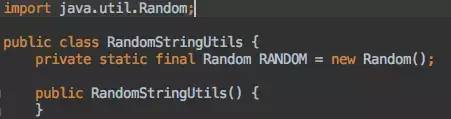RandomStringUtils 类的定义

展开全文java 算法 多线程 Random
• ## Random

千次阅读 2018-02-26 17:38:35
Random模块1、Python中的random模块Random
• Random Erasing是2017年与cutout同时期提出的一种数据增强方法，想法比较简单，即在训练时随机裁剪掉图像的一部分，也可以看作是一种类似dropout的正则化方法。 Random Erasing Data Augmentation paper (arXiv):...数据增强 图像分类 深度学习
• ## java的Random类详解

千次阅读 2021-01-14 14:51:48
Random类 (java.util)Random类中实现的随机算法是伪随机，也就是有规则的随机。在进行随机时，随机算法的起源数字称为种子数(seed)，在种子数的基础上进行一定的变换，从而产生需要的随机数字。相同种子数的Random...
• ## 随机数Random

万次阅读 多人点赞 2020-08-13 17:04:36
Random 一个用于生成随机数的类 具体用法 //创建随机数对象 Random random = new Random(); //随机产生一个int类型取值范围内的数字。 int num1 = random.nextInt(); System.out.println(num1); //产生一...java random 算法
• Random类主要用来生成随机数，本文详解介绍了Random类的用法，希望能帮到大家。Random类 (java.util)Random类中实现的随机算法是伪随机，也就是有规则的随机。在进行随机时，随机算法的起源数字称为种子数(seed)，在...
• 目录前言random.random()random.choice()random.choices()random.getrandbits()random.getstate()random.setstate()random.randint()random.randrange()random.sample()random.seed()random.shuffle()random.uniform...python random
• 文章目录java.util.Randomjava.Security.SecureRandom/dev/random 与 /dev/urandom资料 Java 里提供了一些用于生成随机数的工具类，这里分析一下其实现原理，以及他们之间的区别、使用场景。 java.util.Random ...Java 随机数 强随机数 Random
• Random类主要用来生成随机数，下面列出了他的常用方法和使用案例。 Random类 (java.util) Random类中实现的随机算法是伪随机，也就是有规则的随机。在进行随机时，随机算法的起源数字称为种子数(seed)，在种子数...算法 java 编程语言
• 传奇脚本关于Random机率算法详细说明 说明：Random 是一个随机标签，一般用于判定脚本里，#if的下面，当然这个标签也用在新爆率文件里，可以查看我写的一篇关于爆率的文章，这里就不多讲了 格式：Random 数值(数值与...
• ## Random和random的区别

千次阅读 2020-08-19 15:05:50
Randomrandom的区别，以及他们的优缺点， 使用的区别:       random属于java.lang包下Math类的方法，属于静态方法，不需要创建对象，只用通过类名点的方式直接调用即可,返回值为...java random
• import random random.seed(1) # 随机生成浮点数 print("%04.2f" % random.random()) # 随机生成浮点数 print("%04.2f" % random.uniform(100, 200)) # 随机生成整型 print(random.randint(1, 200)) # 随机生成整型...
• 1. python自带random包： 提供一些基本的随机数产生函数，可满足基本需要 2. numpy.random：提供一些产生随机数的高级函数，满足高级需求 random 介绍 函数 功能 备注 random.random() 生成一个0-1...python 随机数
• Random类(java.util)Random类中实现的随机算法是伪随机，也就是有规则的随机。在进行随机时，随机算法的起源数字称为种子数(seed)，在种子数的基础上进行一定的变换，从而产生需要的随机数字。相同种子数的Random...
• python中random.randint(1,100)随机数中包不包括1在python中的random.randint(a,b)用于生成一个指定范围内的整数。其中参数a是下限，参数b是上限。import random print random.random(0,100)小编知道小编自己演技很...
• Python随机函数库random的使用方法详解前言众所周知，python拥有丰富的内置库，还支持众多的第三方库，被称为胶水语言，随机函数库random，就是python自带的标准库，他的用法极为广泛，除了生成比较简单的随机数外，...
• random.random()用于生成一个0到1的随机符点数: 0 <= n < 1.0 其它random的用法详见[random用法参考]python 开发语言 后端 深度学习 pytorch
• 1python自带的random库 随机生成一个n-m之间的整数： random.randint(1,10) 随机生成一个浮点数： random.random()，什么参数都不需要给，随机生成一个0到1之间的浮点数； random.uniform(1.1,5.4)，随机生成一个...python pycharm 开发语言
• random.random（）中，输出介于0&1之间，不接受任何输入参数 而random.uniform（）接受参数，您可以在其中提交随机数的范围。 a、b. random.random()为您提供一个在[0.0, 1.0)范围内的随机浮点数（因此包括...python
• random库是使用随机数（随机产生的一些数，计算机不会产生随机数）的Python标准库（随Python的解释器而自带的库，不需要安装直接使用import来使用它） 真正的随机数也是在各种复杂条件（并不充分理解或已经超越我们...python 后端
• 我并没有发现np.random.random()与np.random.random_sample()在用法上有什么不同之处，所以它们两个在本质上都是一样的，随机给出设定的size尺寸的位于[0,1)半开半闭区间上的随机数。 代码如下： # -*- coding: utf-...python random numpy
• ## C++ random库

千次阅读 2020-09-11 19:30:44
参考博客 C++11 random库 rand函数的问题 C库函数rand生成的是均匀分布的伪随机数，每个随机数的范围在0和一个系统相关的最大值（至少为32767）之间。 rand函数的问题是：很多程序需要不同范围的随机数，一些应用...c++
• Python内置的random模块提供了生成随机数的方法，使用这些方法时需要导入random模块。import random下面介绍下Python内置的random模块的几种生成随机数的方法。1、random.random() 随机生成 0 到 1 之间的浮点数[0.0...
• np.random.random()函数表示生成随机数组 np.random.random([N,D]) 表示生成N行D列的随机数组，其值在0-1之间取值。 np.random.random([20,2]) 输出： [[0.01092784 0.21841317] [0.39689626 0.35895387] [0....python random numpy
• 当使用numpy中random去产生随机数的时候，会发现这三个函数很相似。 那么它们之间有什么区别呢？ 1. np.random.random() 返回半开放区间[0.0,1.0]中的随机浮点。 与np.random.rand()作用一样，只是参数不同而已。 ...python
• 本篇博客主要为 ...文章目录知识点：python random 库第 1 题：第 2 题：第 3 题：第 4 题：第 5 题：试题仓库地址如下： 知识点：python random 库 第 1 题： 题目难度：1 星 题干（问题描述）： 下述哪个选项python
• 文章目录前言一、Math.random的使用二、Random的使用1.导包三、猜数字游戏的编写总结 前言 今天是五一劳动节，率先在这里祝大家节日快乐. 大学封校了，所以我们该学习还是学习 接下来我们来了解一下Java中随机数的...java idea...